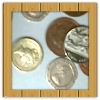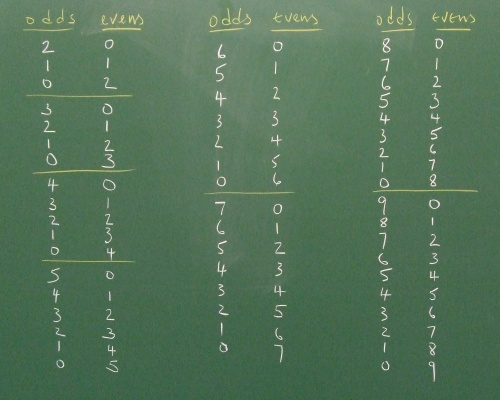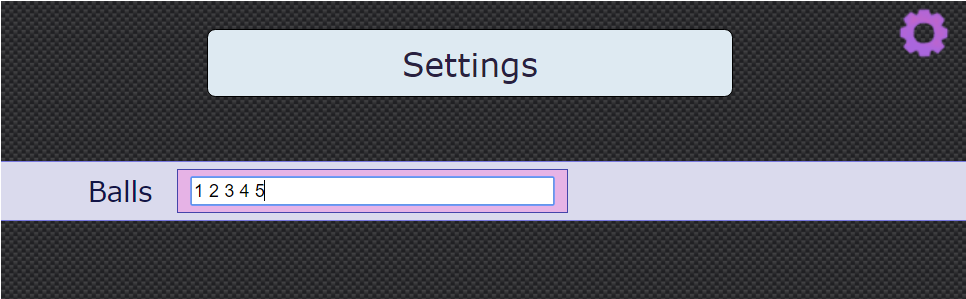#### You may also like### Coin Tossing Games

You and I play a game involving successive throws of a fair coin. Suppose I pick HH and you pick TH. The coin is thrown repeatedly until we see either two heads in a row (I win) or a tail followed by a head (you win). What is the probability that you win?### Win or Lose?

A gambler bets half the money in his pocket on the toss of a coin, winning an equal amount for a head and losing his money if the result is a tail. After 2n plays he has won exactly n times. Has he more money than he started with?A counter is placed in the bottom right hand corner of a grid. You toss a coin and move the star according to the following rules: ... What is the probability that you end up in the top left-hand corner of the grid?

# Odds and Evens Made Fair

### Why do this problem?

This problem offers a meaningful context in which algebraic fractions and tree diagrams can help explain a surprising result in a probability problem. Collaborative working makes it possible for students to tackle an otherwise unmanageable task.

### Possible approach

This problem featured in an NRICH video in June 2020.

This problem follows on from the problem Odds and Evens.
"Imagine you had a bag containing a set of balls with whole numbers on them. You choose two balls, and find the total. Can you find a set of balls where the chance of getting an odd total is equal to the chance of getting an even total?"

Click below for a numerical approach suitable for students without experience working algebraically with tree diagrams.

"How could we go about finding out whether there are any sets that would give a fair game?" One way of organising the search is to draw up a table on the board showing different combinations of odds and evens:Divide the class into groups working on different combinations and ask them to report back. Students could use sample space diagrams or tree diagrams to work out the probability for each case. Then they could record combinations that have been checked on the board with a tick or a cross to show whether they are fair or not.

There will be opportunities while the class are working to stop everyone and share students' insights that will make the job easier. For example: "None of the combinations with zero will work because..." "If 3 odds and 2 evens won't work, 2 odds and 3 evens won't either, because..." "You can't have the same number of evens and odds because..."

Eventually, there will be a sea of crosses on the board and just a few combinations that work (four, if the class have gone up to 9 balls in total). Ask the class to stop and consider what the fair sets have in common. This may lead to some new conjectures about the total number of balls, so organise the class to test the conjectures. Once there is some confirmation about the total number of balls needed for fair games, conjectures can also be made about how these should be split into odds and evens. Students can test examples with large numbers, using the simplified sample space method detailed in the Teachers' notes to Odds and Evens. Draw attention to how valuable it is to work collaboratively as part of a mathematical community, and how difficult it would have been to have reached the same insights working alone.
Finally, invite students to prove their conjectures algebraically. This worksheet outlines one possible proof.

Click below for an algebraic approach using tree diagrams.

"To explore this, imagine there are p balls with odd numbers and q balls with even numbers. Can you work out the probability of an even total or an odd total in terms of p and q?"

Give the class time to work on this. Draw attention to clear methods of representation such as tree diagrams or sample space diagrams. Eventually, students should find that p and q satisfy the equation $(p-q)^2 = p+q$ or equivalent.

"Can we find some values of p and q that satisfy the conditions?" At this point, encourage students to work systematically, and depending on their form for the equation they could set square-number values for $p-q$ and solve the resulting simultaneous equations, or choose a value for p and see whether there is a corresponding integer value for q. Invite students to write up on the board any pairs of numbers p and q they find that work.

"What do you notice about p and q? Can anyone explain why?"
Finally, a little algebra can show why p and q belong to the set of triangle numbers. This worksheet shows one possible proof method.

You might like to use the Odds and Evens Interactivity to show the experimental probabilities for different sets of up to nine numbers. You can click on the purple cog to change the sets of numbers - just list the numbers you want to use, separated by a space, as in the screenshot below:### Key questions

How can you decide if a game is fair?

How can we make this difficult task (of finding a fair game) more manageable?

### Possible support

Take plenty of time to work on the original Odds and Evens problem before trying this extension task.

### Possible extension

The problem In a Box offers another context for exploring exactly the same underlying mathematical structure, and could be used as a follow-up problem a few weeks after working on this one.